Remember, you've got two ways you can double-check this answer to see if it's right: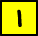Graph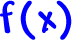and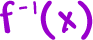on the same graph and see if they're mirror images over the line y = x.(Easy -- since these are both lines.)

Do it!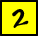Find either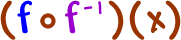or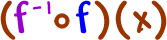(or both for practice!)

*Note:  This is just like ( f o g )( x ), but with different notation.

OK, here's the list of steps:

How to find the inverse of a function:

STEP 1:  Stick a "y" in for the "f(x)."

STEP 2:  Switch the x and y.

STEP 3:  Solve for y.

 STEP 4:  Stick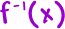in for the "y."

THEN, CHECK IT!